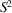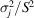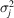International
Tables for
Crystallography
Volume B
Reciprocal space
Edited by U. Shmueli

International Tables for Crystallography (2006). Vol. B. ch. 2.1, p. 195   | 1 | 2 |

## Section 2.1.4.4. Conditions of validity

U. Shmuelia* and A. J. C. Wilsonb

aSchool of Chemistry, Tel Aviv University, Tel Aviv 69 978, Israel, and bSt John's College, Cambridge, England
Correspondence e-mail:  ushmueli@post.tau.ac.il

#### 2.1.4.4. Conditions of validity

| top | pdf |

The above outline of a proof of the central-limit theorem depended on the existence of moments of all orders. The components of structure factors always possess finite moments of all orders, but the existence of moments beyond the second is not necessary for the validity of the theorem and it can be proved under much less stringent conditions. In fact, if all the random variables in equation (2.1.4.19)have the same distribution – as in a homoatomic structure – the only requirement is that the second moments of the distributions should exist [the Lindeberg–Lévy theorem (e.g. Cramér, 1951)]. If the distributions are not the same – as in a heteroatomic structure – some further condition is necessary to ensure that no individual random variable dominates the sum. The Liapounoff proof requires the existence of third absolute moments, but this is regarded as aesthetically displeasing; a theorem that ultimately involves only means and variances should require only means and variances in the proof. The Lindeberg–Cramér conditions meet this aesthetic criterion. Roughly, the conditions are that, the variance of the sum, should tend to infinity and, whereis the variance of the jth random variable, should tend to zero for all j as n tends to infinity. The precise formulation is quoted by Kendall & Stuart (1977, p. 207).

### ReferencesCramér, H. (1951). Mathematical methods of statistics. Princeton University Press.Google ScholarKendall, M. & Stuart, A. (1977). The advanced theory of statistics, Vol. 1, 4th ed. London: Griffin.Google Scholar